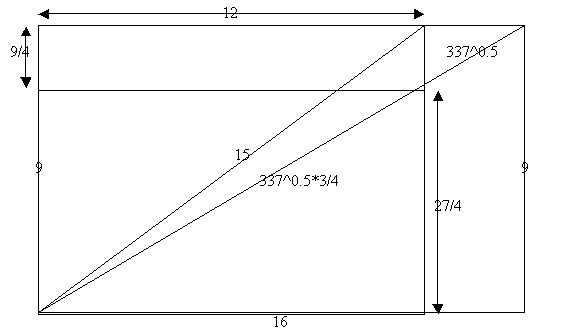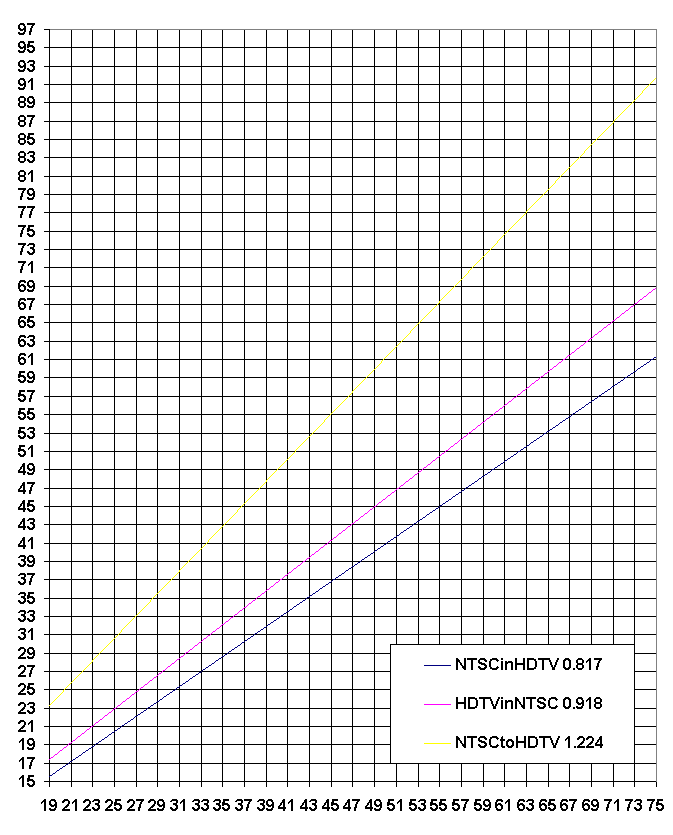# HDTV versus NTSC Screen Size

Reminder: Pythagorean Theorem is a^2 + b^2 = c^2 for right triangles

Standard NTSC aspect ratio is 4:3, forming a 3:4:5 right triangle with diagonal 5.

HDTV aspect ratio is 16:9, the diagonal is SQRT(337) = 18.358Scale factor from HDTV diagonal to viewable NTSC diagonal [NTSCinHDTV]

0.817 = 15/337^0.5

Scale factor from NTSC diagonal to needed HDTV diagonal [NTSCtoHDTV]

1.224 = 337^0.5/15

Scale factor from NTSC diagonal to viewable HDTV diagonal [HDTVinNTSC]

0.918 = 337^0.5/20 = 337^0.5*(3/4)/15

Empty area on HDTV screen viewing full-height NTSC image

¼

Empty area on NTSC screen viewing full-width HDTV image

¼7/18/2002 Gary J. Murakami